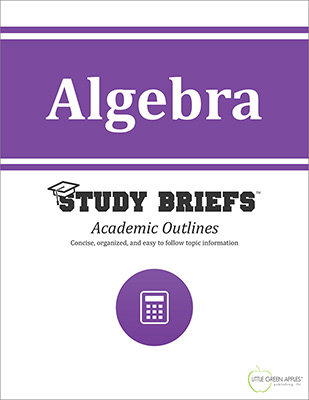# AlgebraLook inside
• Format: eBook
• Edition: 2016
• Publisher: Little Green Apples Publishing LLC

Price: \$4.99

## Description:

The Algebra Study Brief focuses on algebra fundamentals, offering a clear and concise conceptual overview with coherent definitions of important terms highlighted. It is intended as a quick reference for students of higher-level math or as a convenient refresher for lifelong learners interested in a condensed summary of major points. Numerous example formulas and equations are included in an easy-to-read and visual format. This Study Brief emphasizzes basic algebraic elements and practical methods of computation that are needed to achieve a solid foundation of comprehension before moving on to more advanced math concepts. Individual sections cover real numbers, complex numbers, operations, polynomials, linear equations, quadratic equations, radical equations and inequalities. Topics such as sets, notations, intersections, unions, complements, Venn diagrams, polynomials, law of exponents, rational expressions and rational exponents are discussed with guidelines, methods, and sample equations as reference to assist in independent problem solving. Ways of solving inequalities are shown with worked-out examples, including inequalities involving absolute value.

## Topics Covered:

• Introduction
• Real Numbers
• Operations
• Polynomials
• Linear Equations